#### You may also likeThis comes in two parts, with the first being less fiendish than the second. Itâ€™s great for practising both quadratics and laws of indices, and you can get a lot from making sure that you find all the solutions. For a real challenge (requiring a bit more knowledge), you could consider finding the complex solutions.### Discriminating

You're invited to decide whether statements about the number of solutions of a quadratic equation are always, sometimes or never true.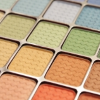This will encourage you to think about whether all quadratics can be factorised and to develop a better understanding of the effect that changing the coefficients has on the factorised form.

# Proving Half-angle Formulae

##### Age 16 to 18Challenge LevelThis resource is from Underground Mathematics.

Take a look at the identities below.

$$\cos^2 \frac{\theta}{2} \equiv \frac{1}{2}(1+\cos \theta) \quad \quad \quad \sin^2 \frac{\theta}{2} \equiv \frac{1}{2}(1-\cos \theta)$$

You may well know enough trigonometric identities to be able to prove these results algebraically, but you could also prove them using geometry. We have provided some diagrams that may help you to prove the result for $\cos^2 \frac{\theta}{2}$. Can you link the diagrams together to form a proof?

You may find it helpful to group the diagrams together in different ways or look for links between pairs of diagrams. You don't need to use all the diagrams in your proof and you may prefer to add some of your own diagrams. The diagrams are available as a print out. There is an extra card in case you'd like to include another diagram in your proof.

cards.pdf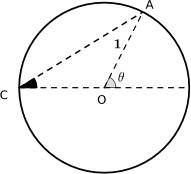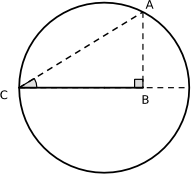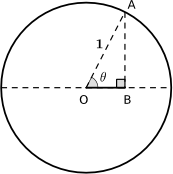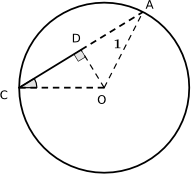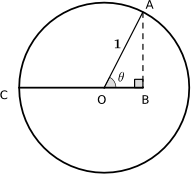Can you prove the result for $\sin^2 \frac{\theta}{2}$ in a similar way?

This is an Underground Mathematics resource.

Underground Mathematics is hosted by Cambridge Mathematics. The project was originally funded by a grant from the UK Department for Education to provide free web-based resources that support the teaching and learning of post-16 mathematics.

Visit the site at undergroundmathematics.org to find more resources, which also offer suggestions, solutions and teacher notes to help with their use in the classroom.